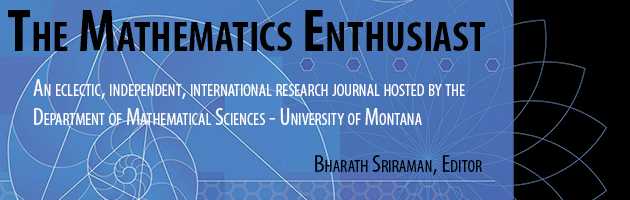•
•8

1-2

#### Abstract

The interconnecting problem approach suggests that often one and the same mathematical problem can be used to teach various mathematical topics at different grade levels. How is this approach useful for the development of mathematical ability and the enrichment of mathematical experiences of all students including the gifted ones? What are the benefits for teachers’ and what would teachers need to implement this approach? What directions would further research on these issues take? The paper discusses these and closely related questions.

I propose that a long-term study of a progression of mathematical ideas revolved around one interconnecting problem is useful for developing a perception of mathematics as a connected subject for all learners. Having a natural appreciation for linking learned material, mathematically-able students exposed to this approach could develop more comprehensive thinking, applicable in many other problem solving situations, such as multiple-solution tasks. Because the problem’s solutions vary in levels of difficulty, as well as conceptual richness, the approach allows teachers to form a strategic vision through a systematic review of various mathematical topics in connection with one problem.

General pedagogical ideas outlined in this paper are supported by discussions of concrete mathematical examples and classroom applications. While individual successful practices of using this approach are known to be taking place, the need for more data collection and interpretation is highlighted.

344

382

COinS